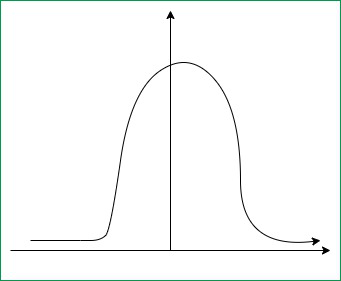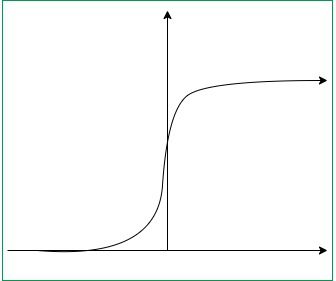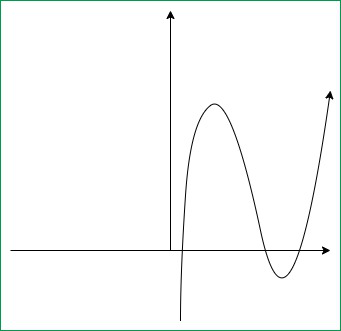Open in App
Not now

# Major Kernel Functions in Support Vector Machine (SVM)

• Difficulty Level : Hard
• Last Updated : 07 Feb, 2022

Kernel Function is a method used to take data as input and transform it into the required form of processing data. “Kernel” is used due to a set of mathematical functions used in Support Vector Machine providing the window to manipulate the data. So, Kernel Function generally transforms the training set of data so that a non-linear decision surface is able to transform to a linear equation in a higher number of dimension spaces. Basically, It returns the inner product between two points in a standard feature dimension.
Standard Kernel Function Equation :Major Kernel Functions :-
For Implementing Kernel Functions, first of all, we have to install the “scikit-learn” library using the command prompt terminal:

    pip install scikit-learn
• Gaussian Kernel: It is used to perform transformation when there is no prior knowledge about data.• Gaussian Kernel Radial Basis Function (RBF): Same as above kernel function, adding radial basis method to improve the transformation.Gaussian Kernel Graph

Code:

## python3

 from sklearn.svm import SVCclassifier = SVC(kernel ='rbf', random_state = 0) # training set in x, y axisclassifier.fit(x_train, y_train)

• Sigmoid Kernel: this function is equivalent to a two-layer, perceptron model of the neural network, which is used as an activation function for artificial neurons.Sigmoid Kernel Graph

Code:

## python3

 from sklearn.svm import SVCclassifier = SVC(kernel ='sigmoid')classifier.fit(x_train, y_train) # training set in x, y axis

• Polynomial Kernel: It represents the similarity of vectors in the training set of data in a feature space over polynomials of the original variables used in the kernel.Polynomial Kernel Graph

Code:

## python3

 from sklearn.svm import SVCclassifier = SVC(kernel ='poly', degree = 4)classifier.fit(x_train, y_train) # training set in x, y axis

Code:

## python3

 from sklearn.svm import SVCclassifier = SVC(kernel ='linear')classifier.fit(x_train, y_train) # training set in x, y axis

My Personal Notes arrow_drop_up